# filters

## Inner reloid through the lattice Gamma ★★

Author(s): Porton

Conjecturefor every funcoid.

Counter-example: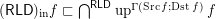for the funcoid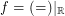is proved in this online article.

Keywords: filters; funcoids; inner reloid; reloids

## Chain-meet-closed sets ★★

Author(s): Porton

Let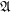is a complete lattice. I will call a filter base a nonempty subsetofsuch that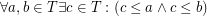.

Definition   A subsetof a complete latticeis chain-meet-closed iff for every non-empty chain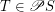we have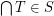.
Conjecture   A subsetof a complete latticeis chain-meet-closed iff for every filter basewe have.

## Co-separability of filter objects ★★

Author(s): Porton

Conjecture   Letandare filters on a setand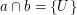. Then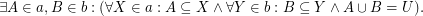See here for some equivalent reformulations of this problem.

This problem (in fact, a little more general version of a problem equivalent to this problem) was solved by the problem author. See here for the solution.

Maybe this problem should be moved to "second-tier" because its solution is simple.

Keywords: filters

## Pseudodifference of filter objects ★★

Author(s): Porton

Letis a set. A filter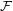(on) is a non-empty set of subsets ofsuch that. Note that unlike some other authors I do not require.

I will call the set of filter objects the set of filters ordered reverse to set theoretic inclusion of filters, with principal filters equated to the corresponding sets. See here for the formal definition of filter objects. I will denotethe filter corresponding to a filter object. I will denote the set of filter objects (on) as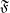.

I will denotethe set of atomic lattice elements under a given lattice element. Ifis a filter object, thenis essentially the set of ultrafilters over.

Problem   Which of the following expressions are pairwise equal for all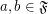for each set? (If some are not equal, provide counter-examples.)
\item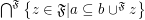;

\item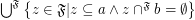;

\item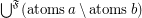;

\item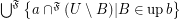.

Keywords: filters; pseudodifference